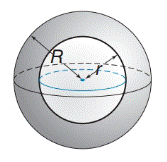Chapter 9.4, Problem 50EElementary Geometry For College St...

7th Edition
Alexander + 2 others
ISBN: 9781337614085

Solutions

Chapter
SectionElementary Geometry For College St...

7th Edition
Alexander + 2 others
ISBN: 9781337614085
Textbook Problem

Derive a formula for the total surface area of the hollow-core sphere.(Note: Include both interior and exterior surface areas.)To determine

To find:

The formula for the total surface area of the hollow-core sphere.

Explanation

Approach:

A sphere is a three dimensional solid figure, which is made up of all the points in space, which lie at a common distance, called the radius, from a fixed point called the center of the sphere. The surface area of a sphere S=4πr2, where r is the radius of the sphere.

All solid bodies occupy space. The measure of occupied space is called the volume of the object. Volume of a sphere is V=43πr3.

Concentric spheres have the same center but different radii.

Calculation:

Consider a hollow-core sphere.

Length of the radius of the inside sphere =r

Length of the radius of the outside sphere =R.

To find the total surface area of the hollow-core sphere, we calculate the surface area of both the concentric spheres and add them up.

Total surface area of hollow-core sphere =Surface area o

Still sussing out bartleby?

Check out a sample textbook solution.

See a sample solution

The Solution to Your Study Problems

Bartleby provides explanations to thousands of textbook problems written by our experts, many with advanced degrees!

Get Started

II. Operating Leverage and Business Risk Once you have determined the break-even poi...

Mathematical Applications for the Management, Life, and Social Sciences

In Exercises 914, evaluate the expression. 9. (94)3/2

Applied Calculus for the Managerial, Life, and Social Sciences: A Brief Approach

Prove each identity. cos()csc()tan()=1

Trigonometry (MindTap Course List)